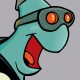ROS Resources: Documentation | Support | Discussion Forum | Index | Service Status | Q&A answers.ros.org

# How to correct the "yaw" behavior of a quadrotor when crossing the angles' limits?!?!

Hi guys,

My quadrotor has a strange behavior (video) when it turns around 180° (the returned value of atan2()). After investigating for hours I 'm pretty sure I found what the problem. Referring to this tutorial as a reference I realized that the angle around the vertical axis (which in my quadrotor is exactly the same structure, see my code here from line 34) , in the tutorial expressed as angle :

tf::createQuaternionMsgFromYaw(angle+M_PI/2);


is simply added by a small quantity calculated at each pass and doesn't take care of the fact that for angles bigger than 180° (or smaller than -180°) the corresponding quaternion has the same value 8or the same orientation if you prefer). In other words, the method tf::createQuaternionMsgFromYaw is going to calculate the correct value even if the angles is crossing those limits.

The nagle in my application jumps about the Z-axis from the positive sector to the negative (and viceversa) because the returned value from atan2() is outputting right values but with the wrong interpretation. As you can see, in the following output (all values are in degree for simplify):

...
Yaw: -164.3665
Yaw: -174.0903
Goal reached
Moving to the 3 waypoint
Yaw: 172.8751
Yaw: 161.7627
Yaw: 153.9304
Yaw: 148.2633
Yaw: 144.0085
...


is clear that the quadrotor must move its nose from -174° to + 172°. But instead of to take the shortest way to to that, it turns counterclockwise along the longest path. I think that this is the normal behavior of tf::createQuaternionMsgFromYaw() which doesn't consider the shortest way to reach the next angle, but it output the corresponding quaternion to that value 8could I have a confirmation about that?)

Now: one could say: "get rid of an absolut coordinate system for your quadrotor and calculate the angle about the Z-axis as a variable, which is going to be changed by small increments every time your code runs a loop...exact like in the tutorial you mentioned!!!"

That's what I thought at the beginning, but I have the problem that all the position and attitude controller for quadrotor are using a fixed coordinate system for their calculation:Resume: I want to correct the behavior of my quadrotor about the Z-axis. To do that I could:

• Rewrite all my controllers for attitude and position to use a body coordinate system and treating the yaw a variable which increments by small changes in orientation without taking care about the limits I said above -> I ve really no idea how to rewrite my code to use such a variable change and I think it would lead to new problems;
• Use two "yaws": one absolut for the controllers and the other one "locally" which is going to be passed to the method tf::createQuaternionMsgFromYaw(). As above...I ve no idea how to implement it in my code;

What would you do guys? Could you me point to the right direction or give my the most used solution ...

edit retag close merge delete

Sort by » oldest newest most votedWhat format you store the angular positions in does not change the wrapping problem on a unit circle. You need to have an algorithm which computes wrapping the appropriate way for your applications.

The angles has some of the most common algorithms for this. In particular I suggest you look at the shortet_angular_distance method.

more